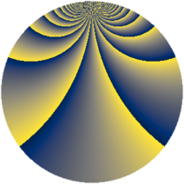# Properties

 Label 1183.2.chLevel $1183$ Weight $2$ Character orbit 1183.ch Rep. character $\chi_{1183}(24,\cdot)$ Character field $\Q(\zeta_{156})$ Dimension $5712$ Sturm bound $242$

# Related objects

## Defining parameters

 Level: $$N$$ $$=$$ $$1183 = 7 \cdot 13^{2}$$ Weight: $$k$$ $$=$$ $$2$$ Character orbit: $$[\chi]$$ $$=$$ 1183.ch (of order $$156$$ and degree $$48$$) Character conductor: $$\operatorname{cond}(\chi)$$ $$=$$ $$1183$$ Character field: $$\Q(\zeta_{156})$$ Sturm bound: $$242$$

## Dimensions

The following table gives the dimensions of various subspaces of $$M_{2}(1183, [\chi])$$.

Total New Old
Modular forms 5904 5904 0
Cusp forms 5712 5712 0
Eisenstein series 192 192 0

## Trace form

 $$5712q - 24q^{2} - 78q^{3} - 20q^{4} - 72q^{5} - 12q^{6} - 54q^{7} - 100q^{8} + 434q^{9} + O(q^{10})$$ $$5712q - 24q^{2} - 78q^{3} - 20q^{4} - 72q^{5} - 12q^{6} - 54q^{7} - 100q^{8} + 434q^{9} - 66q^{10} - 28q^{11} - 86q^{12} - 28q^{14} - 114q^{15} - 248q^{16} - 72q^{17} - 22q^{18} - 70q^{19} + 36q^{20} - 50q^{21} - 48q^{22} - 72q^{23} - 90q^{24} - 26q^{25} - 102q^{26} - 34q^{28} - 104q^{29} + 78q^{30} - 40q^{31} - 136q^{32} - 96q^{33} - 12q^{34} - 62q^{35} - 158q^{36} - 10q^{37} - 78q^{38} + 50q^{39} - 126q^{40} - 18q^{41} - 20q^{42} - 152q^{43} - 72q^{44} - 90q^{45} - 148q^{46} - 36q^{47} - 12q^{48} - 60q^{49} - 114q^{50} - 38q^{51} - 50q^{52} - 30q^{53} - 48q^{54} - 18q^{55} - 28q^{56} - 116q^{57} - 88q^{58} - 72q^{59} - 42q^{60} - 78q^{61} + 36q^{62} - 274q^{63} - 104q^{64} - 24q^{65} - 84q^{66} - 438q^{67} - 72q^{68} - 276q^{69} + 296q^{70} - 62q^{71} + 12q^{72} - 92q^{73} + 396q^{74} - 448q^{75} - 52q^{76} - 52q^{77} - 42q^{78} - 34q^{79} - 90q^{80} - 454q^{81} + 30q^{82} + 66q^{83} + 4q^{84} + 158q^{85} + 316q^{86} - 120q^{87} + 182q^{88} - 48q^{89} + 72q^{90} - 10q^{91} + 84q^{92} - 40q^{93} - 546q^{94} - 20q^{95} - 96q^{96} + 406q^{97} - 164q^{98} - 68q^{99} + O(q^{100})$$

## Decomposition of $$S_{2}^{\mathrm{new}}(1183, [\chi])$$ into newform subspaces

The newforms in this space have not yet been added to the LMFDB.